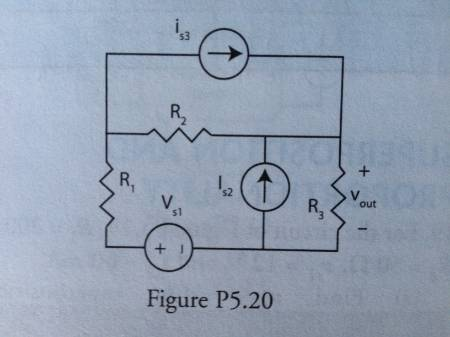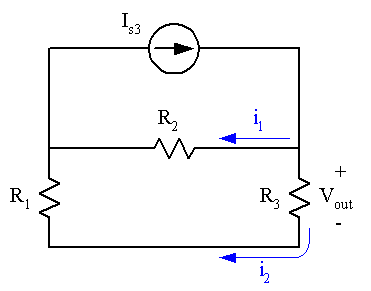# Superposition Problem- Circuits

• Engineering

## Homework StatementFor the above circuit, find the coefficients of the linear relationship vout = a1vs1 + a2is2 + a3is3 by superposition. Then find the power delivered to R3 when vs1 = 100 V, is2 = 2 A, and is3 = 4 A. Given: R1 = 20 Ω, R2 = 60 Ω, and R3 = 20 Ω.

## Homework Equations

Ohm's Law: V = IR

KVL: V1 + V2 + ... Vn = 0 for closed loops

KCL: I1 + I2 + ... In = 0 going in an out of a node

Voltage Division: V1 = Vsource * (R1/(R1+R2))

## The Attempt at a Solution

I think I got the first two coefficients (though I could be incorrect). I found vout1 = vs1*(R3/(R1+R2+R3)) = 0.2Vs1 through voltage division. I'm pretty certain that's correct.

I also found that vout2 = Is2*R3 = 20Is2, though I'm not quite as certain about that.

But I absolutely cannot wrap my head around finding vout3 (when all independent sources are eliminated except Is3). I understand that in that situation, the Is2 branch essentially disappears and we are left with Is3 in parallel with a branch with R2 and a branch with R1 and R3, but we can't combine those since vout3 must stay isolated. I'm not sure what to do.

gneill
Mentor
You have a classic current divider scenario with current Is3 being divided between two branches. You know the total resistance of each of the branches.Alternatively, consider converting Is3 and R2 to their Thevenin equivalent and finding the resulting current and then the potential across R3.

#### Attachments

So I want to use current division to find i2, then multiply that by R3 to get my voltage value?

i2 = Is3*(R2/(R1+R2+R3))
vout3 = i2R3
vout3 = Is3*((R2*R3)/(R1+R2+R3))

After substituting values in, vout3 comes out to be 12. Unfortunately either that or one of my previous solutions is wrong- a later part of the problem states that the total vout must be 100 V when vs1 = 100 V, is2 = 2 A, and is3 = 4 A. And (0.2*100) + (20*2) + (12*4) is 108. Did I go about finding vout3 the wrong way?

gneill
Mentor
Check your work for the coefficient for Is2.